# Rotation of a vector field

(diff) ← Older revision | Latest revision (diff) | Newer revision → (diff)

on a plane

One of the characteristics of a vector field that are invariant under homotopy. Letbe a vector field on a domainof the Euclidean plane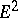and letbe the angle betweenand some fixed direction; the rotation ofwill then be the increment of the anglewhen going around a closed oriented curve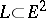along which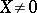, divided by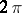. For instance, ifis a smooth curve of class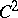, the rotation of the field(or) tangent to(or normal to) alongis equal to the total curvature ofdivided by; ifis a vector field (with or without isolated singular points) on a domain, with Jordan boundary, then the rotation ofon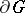is equal to the sum of the indices of the singular points ofin the closure of(cf. Singular point, index of a). The rotation of a vector field remains unchanged during a homotopic deformation ofwhich does not pass through the singular points of.

A generalization consists of the concept of the index of a vector fieldon an-dimensional manifold, at an isolated pointof. It is defined as the degree of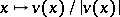, as a mapping from a small sphere aroundto the unit sphere (cf. Degree of a mapping). It is related to the Euler characteristic. See also Poincaré theorem; Kronecker formula.